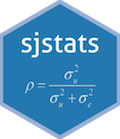sjstats - Collection of Convenient Functions for Common Statistical ComputationsCollection of convenient functions for common statistical computations, which are not directly provided by R’s base or stats packages.

This package aims at providing, first, shortcuts for statistical measures, which otherwise could only be calculated with additional effort (like standard errors, Cronbach’s Alpha or root mean squared errors), or for which currently no functions available.

Second, these shortcut functions are generic (if appropriate), and can be applied not only to vectors, but also to other objects as well (e.g., the Coefficient of Variation can be computed for vectors, linear models, or linear mixed models; the r2()-function returns the r-squared value for lm, glm, merMod, glmmTMB, or lme and other objects).

Most functions of this package are designed as summary functions, i.e. they do not transform the input vector; rather, they return a summary, which is sometimes a vector and sometimes a tidy data frame (where column names follow a common convention). The focus of most functions lies on summary statistics or fit measures for regression models, including generalized linear models, mixed effects models or Bayesian models. However, some of the functions deal with other statistical measures, like Cronbach’s Alpha, Cramer’s V, Phi etc.

The comprised tools include:

• For regression and mixed models: Coefficient of Variation, Root Mean Squared Error, Residual Standard Error, Coefficient of Discrimination, R-squared and pseudo-R-squared values, standardized beta values, p-values
• Especially for mixed models: Design effect, ICC, sample size calculation and convergence tests
• Especially for Bayesian models: Highest Density Interval, region of practical equivalence (rope), Monte Carlo Standard Errors, ratio of number of effective samples, mediation analysis, Test for Practical Equivalence
• Fit and accuracy measures for regression models: Overdispersion tests, accuracy of predictions, test/training-error comparisons, error rate and binned residual plots for logistic regression models
• For anova-tables: Eta-squared, Partial Eta-squared, Omega-squared and Partial Omega-squared statistics

Furthermore, sjstats has functions to access information from model objects, which either support more model objects than their stats counterparts, or provide easy access to model attributes, like:

• model_frame() to get the model frame,
• model_family() to get information about the model family, link functions etc.,
• link_inverse() to get the link-inverse function,
• pred_vars() and resp_var() to get the names of either the dependent or independent variables, or
• var_names() to get the “cleaned” variables names from a model object (cleaned means, things like s() or log() are removed from the returned character vector with variable names.)

Other statistics:

• Cramer’s V, Cronbach’s Alpha, Mean Inter-Item-Correlation, Mann-Whitney-U-Test, Item-scale reliability tests

Documentation

Please visit https://strengejacke.github.io/sjstats/ for documentation and vignettes.

Installation

Latest development build

To install the latest development snapshot (see latest changes below), type following commands into the R console:

library(devtools)
devtools::install_github("strengejacke/sjstats")

Please note the package dependencies when installing from GitHub. The GitHub version of this package may depend on latest GitHub versions of my other packages, so you may need to install those first, if you encounter any problems. Here’s the order for installing packages from GitHub:

Officiale, stable release

To install the latest stable release from CRAN, type following command into the R console:

install.packages("sjstats")

Citation

In case you want / have to cite my package, please use citation('sjstats') for citation information.